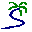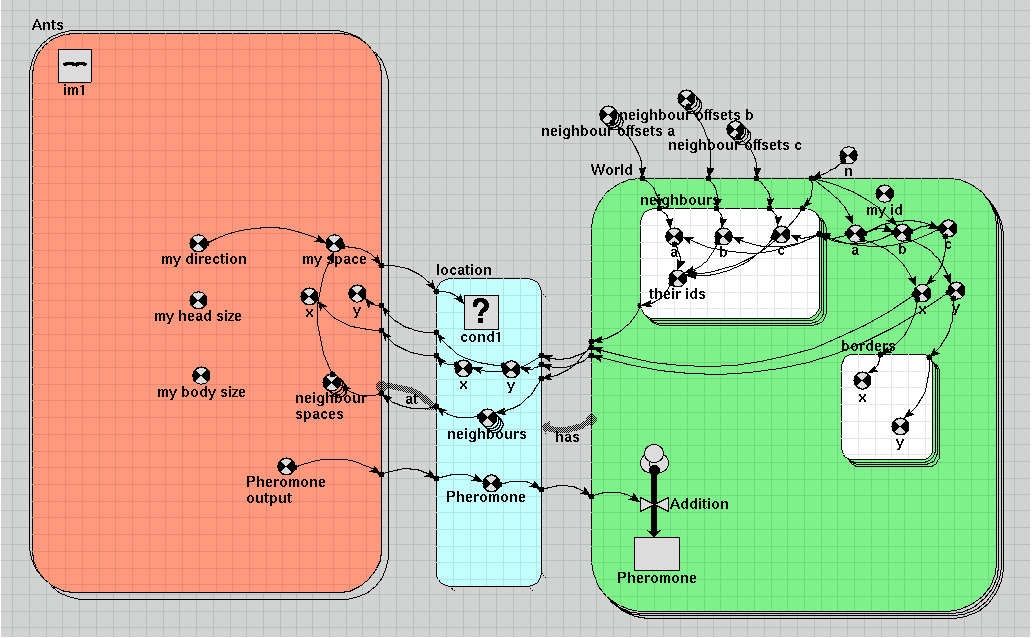# Model entry

Animal movement and interaction with their environment - Model catalogue - Simulistics.com

 Search Simulistics Model catalogue Listed by keyword Listed by ID Listed by title Listed by date added

# Animal movement and interaction with their environment

Model : AntsWorld
Simile version : 4.0
Keywords : Hexagonal grid ; Association submodel ; Movement ; One sided enumeration ; Spatial modelling ;

## Description

In this model, a population of animals (called ants) is created and the individuals move around at random. The area in which they move is represented by a grid of hexagons.

An association model is created to relate each individual to the hexagon it currently occupies. The technique of one-sided enumeration is used to make this association; it is vital that it happens efficiently, because it changes each time step. In this case the position of each ant is used to determine the index of the submodel instance for the hexagon containing it.

Having this association allows the ant to interact with their immediate environment. In this model, the interaction is simple, and one-way; the ants deposit a 'pheromone' which collects in each hexagonal patch. Since all the ants are in the middle of their area when they appear, and subsequently wander off at random, the pheromone concentration is greatest in the centre (see diagrams).

This prototypical model could easily be enhanced to include more aspects of real ant behaviour. The association betweeen ants and their environment could be made to include neighbouring hexagons as well as those actually occupied, and ants could be made to respond to levels of pheromone in the cells surrounding their own, as well as the presence of other ants.

## Files

### Model file

Click on the icon to download the model file. (You will need Simile to examine and run the model. A free evaluation version is available from the products page.)

Some browsers may attempt to display the model file, rather than open it in Simile; in this case, use the browser back button to return to this page, and use the context menu (invoked by right-clicking on the link) to save the target file to disk.antsworld.sml The 'ants world' modelantsworld.shf Helper state file including locations of ants and distribution of pheromone

## Diagram## Equations

```Equations in antsworld

Variable   n
n = int(sqrt(size(World)))

Variable   neighbour offsets a
neighbour offsets a = [0,1,1,0,-1,-1]

Variable   neighbour offsets b
neighbour offsets b = [1,0,-1,-1,0,1]

Variable   neighbour offsets c
neighbour offsets c = [-1,-1,0,1,1,0]

Equations in Ants

Immigration   im1
im1 =  0.1

Variable   Pheromone output
Pheromone output = 1

Variable   my body size
my body size = 2

Variable   my direction
my direction = ceil(rand_var(0,6))

Variable   my space
my space = infront=element(last([neighbour_spaces]),my_direction),if time()==init_time() then 5102 elseif infront>0 then infront else prev(1)
Where:
my_direction=my direction
[neighbour_spaces]=neighbour spaces

Variable   neighbour spaces
neighbour spaces = [neighbours_at]
Where:
[neighbours_at]= ../location/neighbours (to Ants in at)

Variable   x
x = x_at
Where:
x_at= ../location/x (to Ants in at)

Variable   y
y = y_at
Where:
y_at= ../location/y (to Ants in at)

Equations in location

Condition   cond1
cond1 = index(1) is my_space_at
Where:
my_space_at= ../Ants/my space (from Ants in at)

Variable   Pheromone
Pheromone = Pheromone_output_at
Where:
Pheromone_output_at= ../Ants/Pheromone output (from Ants in at)

Variable   neighbours
neighbours = [their_ids_has]
Where:

[their_ids_has]= ../World/neighbours/their ids (from World in has)

Variable   x
x = x_has
Where:
x_has= ../World/x (from World in has)

Variable   y
y = y_has
Where:
y_has= ../World/y (from World in has)

Equations in World

Compartment   Pheromone
Initial value = 0
Rate of change =  + Addition

Where:
{Pheromone_has}= ../location/Pheromone (to World in has)

Variable   a
a = ceil(index(1)/n)-ceil(n/2)
Where:
n= ../n

Variable   b
b = index(1)-n*a-ceil(n*n/2)
Where:
n= ../n

Variable   c
c = -a-b

Variable   my id
my id = index(1)

Variable   x
x = 50+(a-c)* 0.866

Variable   y
y = 50+b* 1.5

Equations in borders

Variable   x
x = off=sqrt(3/4),x+element([0,off,off,0,-off,-off],index(1))
Where:
x= ../x

Variable   y
y = y+element([1, 0.5, -0.5,-1, -0.5, 0.5],index(1))
Where:
y= ../y

Equations in neighbours

Variable   a
a = a+element([neighbour_offsets_a],index(1))
Where:
a= ../a
[neighbour_offsets_a]= ../../neighbour offsets a

Variable   b
b = b+element([neighbour_offsets_b],index(1))
Where:
b= ../b
[neighbour_offsets_b]= ../../neighbour offsets b

Variable   c
c = c+element([neighbour_offsets_c],index(1))
Where:
c= ../c
[neighbour_offsets_c]= ../../neighbour offsets c

Variable   their ids
their ids = if all([a,b,c]> - (n/2)) and all([a,b,c]Results|This image shows the distribution of pheromone over the grid are after 3000 time units:|